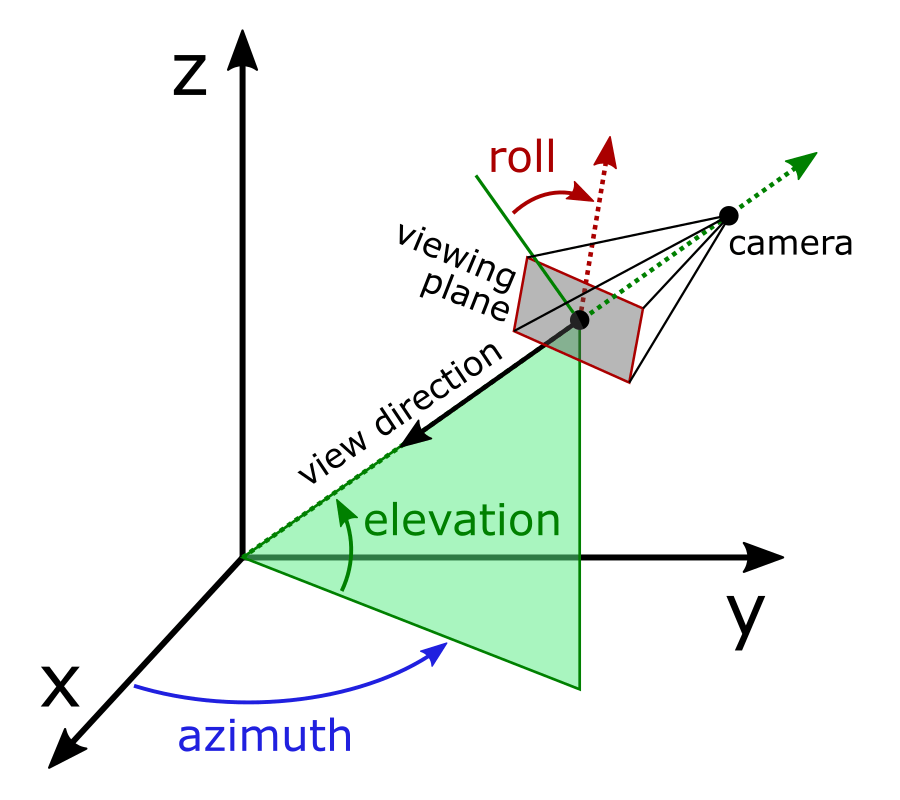# mplot3d View Angles#

## How to define the view angle#

The position of the viewport "camera" in a 3D plot is defined by three angles: elevation, azimuth, and roll. From the resulting position, it always points towards the center of the plot box volume. The angle direction is a common convention, and is shared with PyVista and MATLAB (though MATLAB lacks a roll angle). Note that a positive roll angle rotates the viewing plane clockwise, so the 3d axes will appear to rotate counter-clockwise.Rotating the plot using the mouse will control only the azimuth and elevation, but all three angles can be set programmatically:

```import matplotlib.pyplot as plt
To look directly at the primary view planes, the required elevation, azimuth, and roll angles are shown in the diagram of an "unfolded" plot below. These are further documented in the `mplot3d.axes3d.Axes3D.view_init` API.